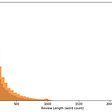# Showcase: Quotes classification

`{  "Quote": "Don't cry because it's over, smile because it happened.",  "Author":"Dr. Seuss",  "Tags":[ "attributed-no-source",  "cry",  "crying", ...],  "Popularity": 0.15566615566615566,  "Category":"life"}`

# Install and import libraries

`!pip install torch!pip install numpy!pip install pandas!pip install matplotlib!pip install seaborn!pip install sklearn!pip install transformers!pip install pytorch-lightning`
`# Import all librariesimport pandas as pdimport numpy as npimport reimport os# Huggingface transformersimport transformersfrom transformers import BertModel,BertTokenizer,AdamW, get_linear_schedule_with_warmupimport torchfrom torch import nn ,cudafrom torch.utils.data import DataLoader,Dataset,RandomSampler, SequentialSamplerimport pytorch_lightning as plfrom pytorch_lightning.callbacks import ModelCheckpointfrom sklearn.model_selection import train_test_splitfrom sklearn.metrics import accuracy_score, precision_recall_fscore_supportimport seaborn as snsfrom pylab import rcParamsimport matplotlib.pyplot as pltfrom matplotlib import rc%matplotlib inlineis_gpu_available = torch.cuda.is_available()device = torch.device("cuda:0" if is_gpu_available else "cpu")if is_gpu_available:  !nvidia-smi`

2. Scroll down to the “API” section and click “Create New API Token”. This will download a file kaggle.json.
`"""The Kaggle dataset path"""KAGGLE_DATASET ='akmittal/quotes-dataset'!pip install -q kagglefrom google.colab import filesfiles.upload()!pip install -q kaggle!mkdir -p ~/.kaggle!mv kaggle.json ~/.kaggle/!ls ~/.kaggle!chmod 600 /root/.kaggle/kaggle.json!kaggle datasets download "{KAGGLE_DATASET}"!mkdir  /content/dataset!unzip -q /content/quotes-dataset.zip -d /content/dataset!ls /content/dataset`

`import pandas as pddf = pd.read_json('/content/dataset/quotes.json')# Take only the required attributes and discard the othersdf = df[['Quote','Tags']]# Drop the duplicates records into our datasetdf = df.drop_duplicates(['Quote'])print(f'The data frame contains {len(df)} records.')df.head(6)`

# Normalize dataset

`df.Tags = df.Tags.transform(lambda tags: [tag.lower().strip() for tag in tags])tags = [element for list_ in df.Tags for element in list_]tags = [tag.lower().strip() for tag in tags]print(f'There are {len(tags)} tags.')# There are 215664 tags.`
`classes = pd.Series(tags).value_counts()[:15].indexclasses = list(set(classes))classes.sort()df['Tags'] = df.Tags.transform(lambda tags: list(set(tags).intersection(classes)))df = df[df.Tags.transform(lambda tags: len(tags)>0)]print(f'We will only consider the following tags: {classes}.')print(f'The data frame contains {len(df)} records with one or more tags.')`
`from sklearn.preprocessing import MultiLabelBinarizermlb = MultiLabelBinarizer()df = df.join(pd.DataFrame(mlb.fit_transform(df.pop('Tags')),                          columns=mlb.classes_,                          index=df.index))df.head(n=6)`

# Splitting dataset into train, test, and validation data

`df = df[:2000]`
`RANDOM_STATE = 167train_data,temp_data = train_test_split(    df,    test_size=.3,     random_state=RANDOM_STATE,    shuffle=True,)test_data, val_data = train_test_split(    temp_data,     test_size=0.5,     random_state=RANDOM_STATE,     shuffle=True,)print(f' - dataset for training model: {train_data.shape}.')print(f' - dataset for validate trained model: {val_data.shape}.')print(f' - dataset for test the model {test_data.shape}.')`

# Preparing the Dataset and DataModule

`class QuoteTagDataset (Dataset):  def __init__(self, data, tokenizer, max_len):      self.tokenizer = tokenizer      self.data      = data      self.max_len   = max_len        def __len__(self):      return len(self.data)    def __getitem__(self, item_idx):      item   = self.data.iloc[item_idx]      quote  = item['Quote']      labels = item[classes]      inputs = self.tokenizer.encode_plus(          quote,          None,          max_length= self.max_len,          padding = 'max_length',          add_special_tokens=True,          return_token_type_ids= False,          return_attention_mask= True,          truncation=True,          return_tensors = 'pt'        )            input_ids = inputs['input_ids'].flatten()      attn_mask = inputs['attention_mask'].flatten()            return {          'input_ids': input_ids ,          'attention_mask': attn_mask,          'label': torch.tensor(labels, dtype=torch.float)          }class QuoteTagDataModule (pl.LightningDataModule):        def __init__(self, train_data, val_data, test_data,tokenizer,train_batch_size=8, val_batch_size=8, test_batch_size=8, max_token_len=150):        super().__init__()        self.train_data = train_data        self.test_data  = test_data        self.val_data   = val_data        self.tokenizer = tokenizer        self.train_batch_size = train_batch_size        self.test_batch_size = test_batch_size        self.val_batch_size = val_batch_size        self.max_token_len = max_token_len    def setup(self):        self.train_dataset = QuoteTagDataset(data=self.train_data, tokenizer=self.tokenizer,max_len = self.max_token_len)        self.val_dataset  = QuoteTagDataset(data=self.val_data,tokenizer=self.tokenizer,max_len = self.max_token_len)        self.test_dataset  = QuoteTagDataset(data=self.test_data,tokenizer=self.tokenizer,max_len = self.max_token_len)                    def train_dataloader(self):        return DataLoader (self.train_dataset, batch_size = self.train_batch_size, shuffle = True , num_workers=0)    def val_dataloader(self):        return DataLoader (self.val_dataset,batch_size=self.val_batch_size)    def test_dataloader(self):        return DataLoader (self.test_dataset,batch_size=self.test_batch_size)# Initialize the Bert tokenizerBERT_MODEL_NAME = 'bert-base-cased'Bert_tokenizer = BertTokenizer.from_pretrained(BERT_MODEL_NAME)# Initialize the parameters that will be use for trainingTRAIN_BATCH_SIZE = 8TEST_BATCH_SIZE  = 8VAL_BATCH_SIZE   = 8MAX_LEN          = 128# Instantiate and set up the data_moduledata_module = QuoteTagDataModule(train_data,val_data,test_data,Bert_tokenizer,TRAIN_BATCH_SIZE,VAL_BATCH_SIZE,TEST_BATCH_SIZE,MAX_LEN)data_module.setup()`

# Train the Model

`class QuoteTagClassifier(pl.LightningModule):        def __init__(self, n_classes=15, steps_per_epoch=None, n_epochs=3, lr=2e-5 ):        super().__init__()        self.bert = BertModel.from_pretrained(BERT_MODEL_NAME, return_dict=True)        self.classifier = nn.Linear(self.bert.config.hidden_size,n_classes) # outputs = number of labels        self.steps_per_epoch = steps_per_epoch        self.n_epochs = n_epochs        self.lr = lr        self.criterion = nn.BCEWithLogitsLoss()            def forward(self,input_ids, attn_mask):        output = self.bert(input_ids = input_ids ,attention_mask = attn_mask)        output = self.classifier(output.pooler_output)        return output            def training_step(self,batch,batch_idx):        input_ids = batch['input_ids']        attention_mask = batch['attention_mask']        labels = batch['label']                outputs = self(input_ids,attention_mask)        loss = self.criterion(outputs,labels)        self.log('train_loss',loss , prog_bar=True,logger=True)                return {"loss" :loss, "predictions":outputs, "labels": labels }    def validation_step(self,batch,batch_idx):        input_ids = batch['input_ids']        attention_mask = batch['attention_mask']        labels = batch['label']                outputs = self(input_ids,attention_mask)        loss = self.criterion(outputs,labels)        self.log('val_loss',loss , prog_bar=True,logger=True)                return loss    def test_step(self,batch,batch_idx):        input_ids = batch['input_ids']        attention_mask = batch['attention_mask']        labels = batch['label']        outputs = self(input_ids,attention_mask)        loss = self.criterion(outputs,labels)        self.log('test_loss',loss , prog_bar=True,logger=True)        return loss            def configure_optimizers(self):        optimizer = AdamW(self.parameters() , lr=self.lr)        warmup_steps = self.steps_per_epoch//3        total_steps = self.steps_per_epoch * self.n_epochs - warmup_steps        scheduler = get_linear_schedule_with_warmup(optimizer,warmup_steps,total_steps)        return [optimizer], [scheduler]N_EPOCHS   = 20LR         = 2e-05steps_per_epoch = len(train_data)//TRAIN_BATCH_SIZEmodel = QuoteTagClassifier(n_classes=len(classes), steps_per_epoch=steps_per_epoch,n_epochs=N_EPOCHS,lr=LR)`
`# Instantiate the Model Trainertrainer = pl.Trainer(max_epochs = N_EPOCHS , gpus = 1, progress_bar_refresh_rate = 20)# Train the Classifier Modeltrainer.fit(model, data_module)`
`# Evaluate the model performance on the test datasettrainer.test(model,datamodule=data_module)`

# Evaluate Model Performance on Test Set

`from torch.utils.data import TensorDataset# Tokenize all quotes in test_datainput_ids = []attention_masks = []for quote in test_data.Quote:    encoded_quote =  Bert_tokenizer.encode_plus(      quote,      None,      add_special_tokens=True,      max_length= MAX_LEN,      padding = 'max_length',      return_token_type_ids= False,      return_attention_mask= True,      truncation=True,      return_tensors = 'pt'          )        input_ids.append(encoded_quote['input_ids'])    attention_masks.append(encoded_quote['attention_mask'])    # Now convert the lists into tensors.input_ids = torch.cat(input_ids, dim=0)attention_masks = torch.cat(attention_masks, dim=0)labels = torch.tensor(test_data[classes].values)# Create the DataLoader.pred_data = TensorDataset(input_ids, attention_masks, labels)pred_sampler = SequentialSampler(pred_data)pred_dataloader = DataLoader(pred_data, sampler=pred_sampler, batch_size=TEST_BATCH_SIZE)flat_pred_outs = 0flat_true_labels = 0# Put model in evaluation modemodel = model.to(device) # moving model to cudamodel.eval()# Tracking variables pred_outs, true_labels = [], []#i=0# Predict for batch in pred_dataloader:    # Add batch to GPU    batch = tuple(t.to(device) for t in batch)      # Unpack the inputs from our dataloader    b_input_ids, b_attn_mask, b_labels = batch     with torch.no_grad():        pred_out = model(b_input_ids,b_attn_mask)        pred_out = torch.sigmoid(pred_out)        pred_out = pred_out.detach().cpu().numpy()        label_ids = b_labels.to('cpu').numpy()    pred_outs.append(pred_out)    true_labels.append(label_ids)flat_pred_outs = np.concatenate(pred_outs, axis=0)flat_true_labels = np.concatenate(true_labels, axis=0)`

# Predictions of Tags in the Test set

`threshold  = np.arange(0.4,0.51,0.01)threshold`
`# convert probabilities into 0 or 1 based on a threshold valuedef classify(pred_prob,thresh):    y_pred = []    for tag_label_row in pred_prob:        temp=[]        for tag_label in tag_label_row:            if tag_label >= thresh:                temp.append(1)             else:                temp.append(0)        y_pred.append(temp)    return y_predfrom sklearn import metricsscores=[] # Store the list of f1 scores for prediction on each threshold#convert labels to 1D arrayy_true = flat_true_labels.ravel() for thresh in threshold:        #classes for each threshold    pred_bin_label = classify(flat_pred_outs,thresh)     #convert to 1D array    y_pred = np.array(pred_bin_label).ravel()    scores.append(metrics.f1_score(y_true,y_pred))# find the optimal thresholdopt_thresh = threshold[scores.index(max(scores))]print(f'Optimal Threshold Value = {opt_thresh}')`

# Performance Score Evaluation

`#predictions for optimal thresholdy_pred_labels = classify(flat_pred_outs,opt_thresh)y_pred = np.array(y_pred_labels).ravel() # Flattenprint(metrics.classification_report(y_true,y_pred))`
`from sklearn.preprocessing import MultiLabelBinarizermlb = MultiLabelBinarizer()yt = mlb.fit_transform([classes])yt.shapey_pred = mlb.inverse_transform(np.array(y_pred_labels))y_act = mlb.inverse_transform(flat_true_labels)df = pd.DataFrame({'Body':test_data['Quote'],'Actual Tags':y_act,'Predicted Tags':y_pred})df.sample(40)`

# References

--

--

--

## More from Iván Corrales Solera

If tech can change the world... why don't we use it to make it better? https://www.linkedin.com/in/ivan-corrales-solera/

Love podcasts or audiobooks? Learn on the go with our new app.

## Exploring Machine Learning: The beginning## Classifying cats and dogs using CNNs## Text Clustering-The beginning## Starbucks Mobile App Offer Analysis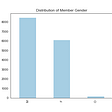## Analysis: Real time super resolution using ESPCN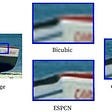## Spell Correction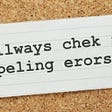## Serving Spark NLP via API (2/3): FastAPI and LightPipelines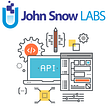## Locally Linear Embedding (LLE) | Data Mining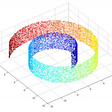## Iván Corrales Solera

If tech can change the world... why don't we use it to make it better? https://www.linkedin.com/in/ivan-corrales-solera/

## Paraphrasing in Natural Language Processing (NLP)## How Transformers transformed NLP## Dependency parsers in Natural Language Processing (NLP)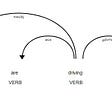## Sentiment Analysis Using NLP and ML